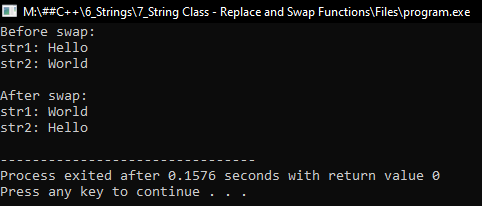# Replace and Swap Functions of String Class in C++

## Replace and Swap Functions of String Class in C++

In this article, I am going to discuss Replace and Swap Functions of String Class in C++ Language with examples. Please read our previous article, where we discussed Append and Insert Functions of String Class in C++ with examples.

##### Replace Function of String Class in C++: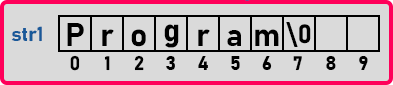Here we have a string “program”. Now we want to replace some characters in this string with some other characters or words. For that, we will write “str1.replace(3, 4, “mise”)”. Now, this function will take 4 characters from the 3rd index onwards and replace them with “mise”.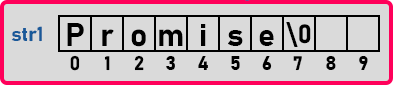This function takes 3 parameters, the first is the index from where we want to replace, the second total no. of character from that index, and the third is the word which we want to replace with. Let us see this in the program.

##### Program for Replace Function of String Class in C++:
```#include <iostream>
#include <string>
using namespace std;
int main()
{
string str = "program";
str.replace (3, 4, "mise");
cout << str << endl;
}
```
###### Output: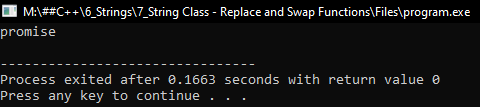Let us look at the next function.

##### erase() Function of String Class in C++:

This function will clear the whole string. It is like clear. We have studied clear in the previous article.

##### Program for erase Function of String Class in C++:
```#include <iostream>
#include <string>
using namespace std;
int main()
{
string str = "program";
str.erase ();
if (str.empty ())
cout << "strign is empty" << endl;
else
cout << str << endl;
}
```
###### Output: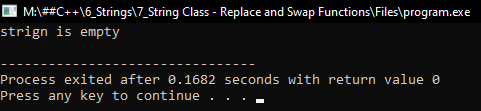##### push_back() Function of String Class in C++:

This function will insert a single character at the end of a string. We can mention only a single character. If we write “str1.push_back(‘z’)” then it will insert ‘z’ at the end of the string.

##### pop_back() Function of String Class in C++:

It will be removing the last character from a string. Let us see both pop_back() and push_back() function in the program.

##### Program for pop_back() and push_back():
```#include <iostream>
#include <cstring>
#include <cstdlib>
using namespace std;
int main()
{
string str = "program";
cout << "String: " << str << endl;
str.push_back ('s');
cout << "Push_back('s'): " << str << endl;
str.pop_back ();
cout << "Pop_back(): " << str << endl;
}
```
###### Output: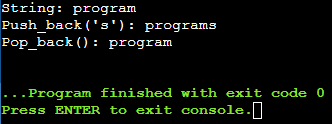So, it is like a stack operation like you can push from the end and pop at the end.

##### swap() Function of String Class in C++:

This function will swap two strings. If we write “str1.swap(str2)” then it will swap the values of str1 and str2. Let’s see this in the program.

##### Program for swap Function of String Class in C++:
```#include <iostream>
#include <cstring>
#include <cstdlib>
using namespace std;
int main()
{
string str1 = "Hello";
string str2 = "World";

cout << "Before swap:" << endl;
cout << "str1: " << str1 << endl;
cout << "str2: " << str2 << endl;

str1.swap (str2);

cout << "\nAfter swap:" << endl;
cout << "str1: " << str1 << endl;
cout << "str2: " << str2 << endl;
}
```
###### Output: